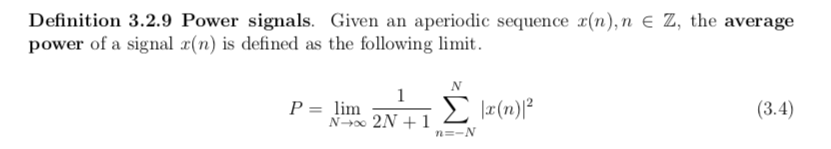Menú

Hello, guys!

Your homework for the next two days is the following.

1. Finish the MATLAB homework. That would be Chapter 2 from Section 2.1 to 2.6. The m-file can be found on this website. Then, do Program 2.7.1 and 2.7.4.
2. Do from  Definition 3.2.8. to Problem 3.2.13. By the way, there is a typo in Equation 3.4. Find the right version below. My apologies for the mistake.Go to top